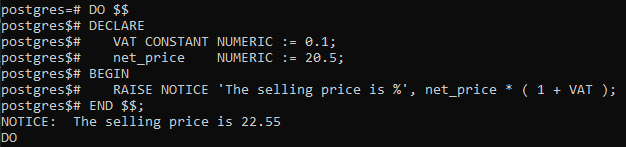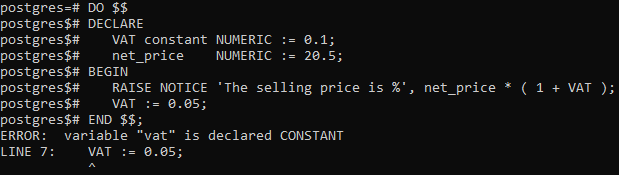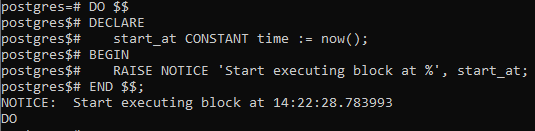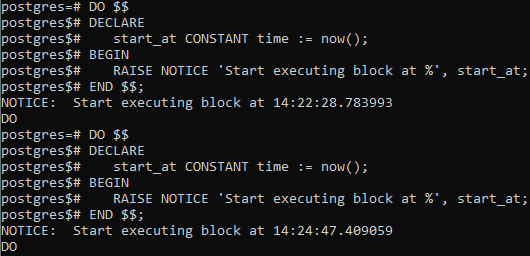Open in App
Not now

# PostgreSQL – Constants

• Last Updated : 28 Aug, 2020

Unlike variables, the value of constants cannot be changed once initialized. The main purpose of the use of constants in PostgreSQL are:

• It makes the query more readable.
• It reduces the maintenance efforts.
```Syntax: constant_name CONSTANT data_type := expression;
```

Let’s analyze the above syntax:

• First, specify the constant name. By convention, it is generally in the uppercase form.
• Second, put the CONSTANT keyword and specify the data type that the constant is associated with.
• Third, initialize a value for the constant.

Example 1:

The following example declares a constant named VAT for valued added tax and calculates the selling price from the net price:

```DO \$\$
DECLARE
VAT CONSTANT NUMERIC := 0.1;
net_price    NUMERIC := 20.5;
BEGIN
RAISE NOTICE 'The selling price is %', net_price * ( 1 + VAT );
END \$\$;
```

Output:Now let’s attempt to change the constant as below:

```DO \$\$
DECLARE
VAT constant NUMERIC := 0.1;
net_price    NUMERIC := 20.5;
BEGIN
RAISE NOTICE 'The selling price is %', net_price * ( 1 + VAT );
VAT := 0.05;
END \$\$;
```

As expected it raises an error as shown below:Example 2:

It is important to note that PostgreSQL evaluates the value for the constant when the block is entered at run-time, not compile-time as shown in the below example:

```DO \$\$
DECLARE
start_at CONSTANT time := now();
BEGIN
RAISE NOTICE 'Start executing block at %', start_at;
END \$\$;
```

Output:PostgreSQL evaluates the NOW( ) function every time we call the block. To prove it, we execute the block again:And got a different result.

My Personal Notes arrow_drop_up